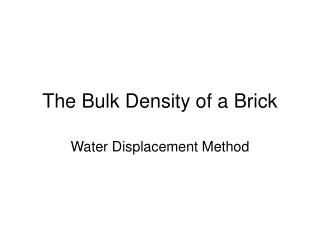Download PresentationThe Bulk Density of a Brick

# The Bulk Density of a Brick - PowerPoint PPT PresentationDownload Presentation## The Bulk Density of a Brick

- - - - - - - - - - - - - - - - - - - - - - - - - - - E N D - - - - - - - - - - - - - - - - - - - - - - - - - - -
##### Presentation Transcript

1. The Bulk Density of a Brick Water Displacement Method

2. Density Density, ρ = Mass Volume The Greek symbol used for density is ρ which is pronounced “ro”.

3. Density Mass is relatively easy as the brick can be weighed in air to give its mass(m1 gm) Mass in air, m1 = 2138.0gm

4. Density Volume is more difficult as the brick is quite a complicated shape, it is not very evenly shaped and it has quite a rough surface.

5. Density However, the Volume can be calculated by weighing the brick in air (m1 gm) and then weighing it fully immersed in water (m2 gm) The mass of water displaced by the brick = Mass in air (m1 gm) – Mass in water (m2 gm) Mass in water m2 = 1030.2gm

6. Density The density of water, ρw, can be assumed to be 1 gm/cm3 Hence, the volume of the brick is numerically the same as the mass of water displaced

7. Density The density can now be calculated Density, ρ = m1 x ρw (m1 – m2)

8. Density Let us put the numbers in for our brick Density, ρ = m1 x ρw (m1 – m2) Density, ρ = 2138.0 x 1 g/cm3 2138.0 – 1030.2 = 1.93 g/cm3 As 1 g/cm3 is the same as 1000 kg/m3, the density can also be stated as 1930 kg/m3 which is nearly 2 Tonnes per m3